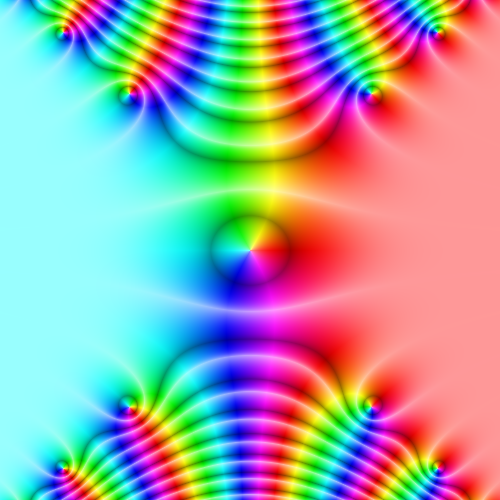Computes the complex error function.

Namespace:  Meta.Numerics.Functions
Assembly:  Meta.Numerics (in Meta.Numerics.dll) Version: 4.1.4Syntax
```public static Complex Erf(
Complex z
)```

#### Parameters

z
Type: Meta.NumericsComplex
The complex argument.

#### Return Value

Type: Complex
The value of erf(z).Remarks

This function is the analytic continuation of the error function (Erf(Double)) to the complex plane.

The image below shows the complex error function near the origin, using domain coloring.The complex error function is entire: it has no poles, cuts, or discontinuities anywhere in the complex plane.

For pure imaginary arguments, erf(z) reduces to the Dawson integral (Dawson(Double)).

Away from the origin near the real axis, the real part of erf(z) quickly approaches ±1. To accurately determine the small difference erf(z) ∓ 1 in this region, use the Faddeeva(Complex) function. Away from the origin near the imaginary axis, the magnitude of erf(z) increases very quickly. Although erf(z) may overflow in this region, you can still accurately determine the value of the product erf(z) exp(z2) using the Faddeeva(Complex) function.See Also